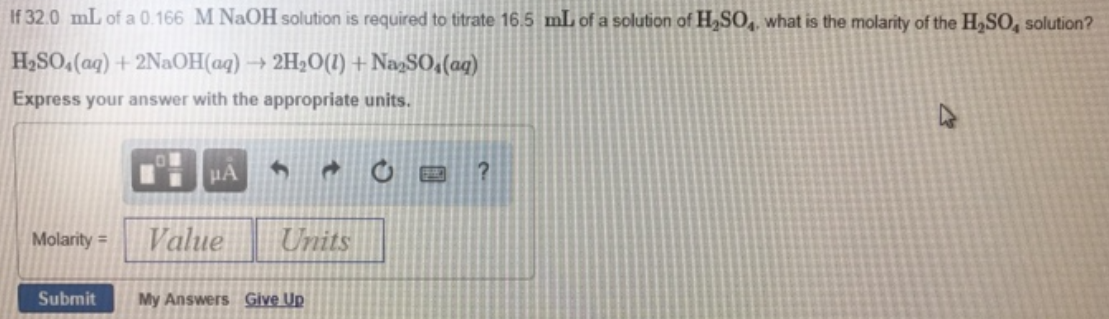# Problem: If 32.0 mL of a 0.166 M NaOH solution is required to titrate 16.5 mL of a solution of H2SO4, what is the molarity of the H2SO4 solution? H2SO4 (aq) + 2NaOH (aq) → 2H2O (l) + Na2SO4 (aq) Express your answer with the appropriate units.

###### FREE Expert Solution
81% (135 ratings)###### Problem Details

If 32.0 mL of a 0.166 M NaOH solution is required to titrate 16.5 mL of a solution of H2SO4, what is the molarity of the H2SO4 solution?

H2SO4 (aq) + 2NaOH (aq) → 2H2O (l) + Na2SO(aq)